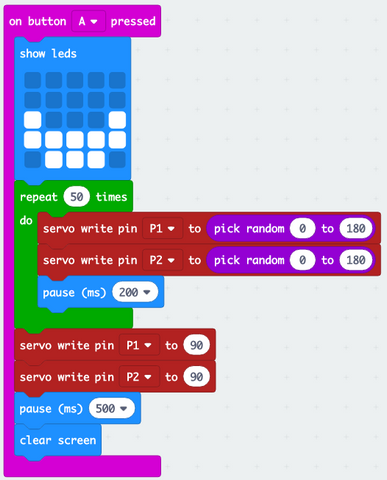# Animatronic Head Kit for micro:bit

\$53.49
SKU 3396

This kit provides everything you need (apart from a micro:bit and batteries) to make a talking animatronic head. Its eyes move and the head speaks from a MonkMakes Speaker for micro:bit.

Makeblock Software

MicroPython Software

Here are a number of coding examples using both block-based programming and MicroPython.

Example 1: Moving eyes forwardsExample 2: Crazy EyesExample 3: Robot BeepingExample 4: Talking Robot

Copy and paste the code below into the MicroPython editor.

`from microbit import *` `import random, speech` `sentences = [` `"Hello my name is Mike",` `"What is your name",` `"I am looking at you",` `"Exterminate exterminate exterminate",` `"Number Five is alive",` `"I cant do that Dave",` `"daisee daisee give me your answer do"` `]` `lips0 = Image("00000:"` `              "00000:"` `              "99999:"` `              "00000:"` `              "00000")` `lips1 = Image("00000:"` `              "00900:"` `              "99099:"` `              "00900:"` `              "00000")` `lips2 = Image("00000:"` `              "09990:"` `              "99099:"` `              "09990:"` `              "00000")` `lips = [lips0, lips1, lips2]` `def set_servo_angle(pin, angle):` `duty = 26 + (angle * 51) / 90` `pin.write_analog(duty)` `def speak(sentence):` `words = sentence.split()` `for i in range(0, len(words)):  ``display.show(random.choice(lips))` `speech.say(words[i])` `display.show(lips0)` `def act():` `angle = random.randint(0, 180)` `set_servo_angle(pin1, angle)` `set_servo_angle(pin2, angle)` `sleep(300)` `speak(random.choice(sentences))` `set_servo_angle(pin1, 90)` `set_servo_angle(pin2, 90)` `sleep(1000)` `base_z = 0` `while True:` `new_z = abs(accelerometer.get_z())` `if abs(new_z - base_z) > 20:` `base_z = new_z` `act()` `if random.randint(0, 1000) == 0: # say something 1 time in 1000` `act() ` `sleep(100)`